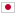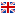Contest Duration: - (local time) (300 minutes) Back to Home
K - Short LIS/Time Limit: 2 sec / Memory Limit: 1024 MB

Score : 100 points

### Problem Statement

You are given three integers N, A, and B.

Let P=(P_0,P_1,...,P_{N-1}) be a permutation of (0,1,...,N-1). P is said good if and only if it satisfies all of the following conditions:

• The length of a longest increasing subsequence of P is at most 2.
• P_A = B

Count the number of good permutations modulo 10^9+7.

### Constraints

• 1 \leq N \leq 10^6
• 0 \leq A \leq N-1
• 0 \leq B \leq N-1

### Input

Input is given from Standard Input in the following format:

N A B


### Output

Print the number of good permutations modulo 10^9+7.

### Sample Input 1

3 0 0


### Sample Output 1

1


The only good permutation is (0,2,1).

### Sample Input 2

12 2 3


### Sample Output 2

5390


### Sample Input 3

10000 9875 5431


### Sample Output 3

135608808